# Comparison between the three theoretical cycles

To compare cycle just discussed, it is necessary to refer some of the factors whose value depends on the shape and surface, such as: the compression ratio, the maximum pressure, the amount of heat supplied and the heat subtracted useful work.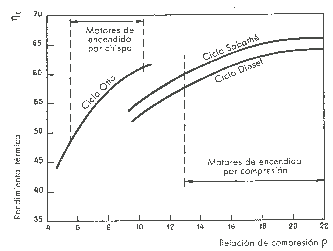The following figure are plotted the curves of the ideal thermal performance variations by varying the ratio of co

RINTING for three cycles: Otto, Diesel and Sabathé. For Diesel cycles and Sabathé has chosen a constant pressure ratio combusted equal to 2.

As shown in the figure, the thermal efficiencies of the theoretical cycles grow by increasing the compression ratio. For a particular such relationship, the Otto cycle gives better performance, while the Diesel cycle performance becomes lower. Must be considered, however, that for Diesel engines, the compression ratio varies between 14 and 22, whereas, for spark ignition engines, generally does not exceed the value of 10, in order to avoid detonation.

Therefore, the diesel engine has a thermal efficiency than gasoline engine.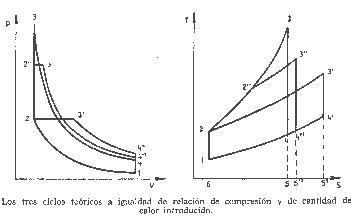In the second figure compares coordinates TS PV and the three cycles, a ratio equal compresióny heat quantity supplied. All cycles begin at the same condition 1 and has the same adiabatic compression of 1 to 2.

In order that the amount of heat supplied is the same in all three cases, coordinates surfaces TS, 2'' 3'' 5'' 6 2 2 ' ', 2 3' 5 '6 2 and 2 3 5 6 2 must be equal.

Given that heat is removed at the same specific volume, according to the processing line between states 4 and 1, the amount of heat subtracted displayed for each cycle, surface itself below the line 4-1 in the diagram TS. As the heat supplied is the same for each cycle, the cycle is higher thermal efficiency is one in which less heat escapes, ie the Otto cycle, to which the surface which represents the amount Heat subtracted TS is defined by coordinates points 4 5 6 1 4.

Note that the maximum temperature in presióny Otto cycle are substantially higher than in the other two runs. While this comparison is purely theoretical, serves to demonstrate that the cycle provides the greatest expansion of the fluid after the heat introduction phase is the highest performance.

Will indicate for the various cycles with rE the ratio between the volumes at the end of the career of expansióny end of the heat introduction phase.

For Otto cycle is: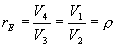while for the diesel cycle are: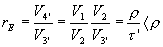We therefore allow the expansion ratio thus defined as the ratio of cycle performance.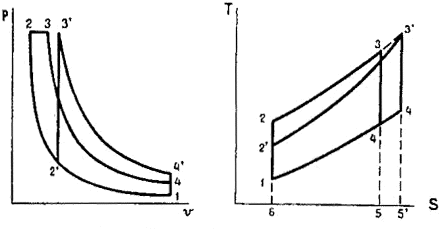In the left figure, the comparison between the theoretical Otto Cycle, Diesel is made equal to maximum pressure and quantity of heat supplied. In these conditions the surfaces 2 3 5 6 2 and 2 '3' 5 '6' 2 'must be equal.

Diesel cycle is one in which subtracts the less heat, and as the surface which represents the heat is subtracted 4 5 6 1 4 it is, therefore, that in these conditions the Diesel cycle is the highest performance.

In this case it is evident that the cycle that allows greater expansion of the fluid after combustion is one that has the highest thermal efficiency.

valoración: 2.8 - votos 13

Last review: February 1, 2016